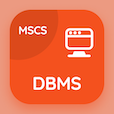Online CS Degree Courses

Computer Networks MCQs

Computer Networks MCQ - Topics

Learn Spread Spectrum Multiple Choice Questions (MCQ Quiz), Spread Spectrum quiz answers PDF to learn computer networks course for computer networks online classes. Bandwidth Utilization: Multiplexing and Spreading Multiple Choice Questions and Answers (MCQs), Spread Spectrum quiz questions for information and communication technology. Spread Spectrum Book PDF: digital signal service, network multiplexing, analog transmission, multiplexers test prep to learn free online courses.

"A pseudorandom code generator that creates a K-bit pattern for every hopping period is known as" MCQ PDF: spread spectrum App APK with hopping, carrier signals, frequency synthesizer, and pseudorandom noise choices for information and communication technology. Study spread spectrum quiz questions for merit scholarship test and certificate programs for top computer science schools in the world.

## MCQs on Spread Spectrum Quiz

MCQ: A pseudorandom code generator that creates a K-bit pattern for every hopping period is known as

hopping
carrier signals
frequency synthesizer
pseudorandom noise

MCQ: In Frequency Hopping Spread Spectrum (FHSS), the sender and receiver can have privacy if the hopping period is

short
long
zero
infinity

MCQ: Institute of Electrical and Electronics Engineers (IEEE) 802.11 Direct Sequence Spread Spectrum (DSSS) uses the data rate of

1 or 2 Mbps
6 to 54 Mbps
5.5 and 11 Mbps
2 and 54 Mbps

MCQ: Direct Sequence Spread Spectrum (DSSS) technique expands the bandwidth of a signal by replacing each data bit with

n+1 bits
n-1 bits
n bits
nx1 bits

MCQ: What is the minimum number of bits in a PN sequence if we use Frequency Hopping Spread Spectrum (FHSS) with a channel bandwidth of B =4 KHz and Bss =100 kHz?

0 bits
5 bits
10 bits
15 bits

### More Quizzes on Computer Networks Book